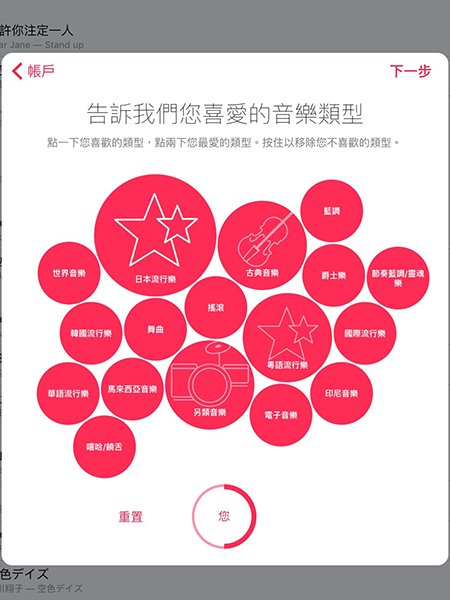#DAY 27
1
Modern Web

## 氣泡圖## pack

``````let pack = d3
.pack()
.size([width, height])
``````

## hierarchy

``````const data = [
{
title: '日本流行樂',
likes: 40,
},
{
title: '古典音樂',
likes: 10,
},
]

let rootNode = d3.hierarchy(dataObj)
const hierarchical_data = rootNode.data
``````

``````const data = [
{
title: '日本流行樂',
likes: 40,
},
{
title: '古典音樂',
likes: 10,
},
]

const dataObj = {
children: data
}

let rootNode = d3.hierarchy(dataObj)
rootNode = rootNode.children
``````

## 繪製流程

``````const width = document.querySelector(`#\${root}`).clientWidth,
height = width

const svg = d3
.select(document.querySelector(`#\${root}`))
.append('div')
.attr('class', 'bubble')
.style('display', 'block')
.append('svg')
.attr('width', width)
.attr('height', height)
``````

``````let pack = d3
.pack()
.size([width, height])
``````

``````let dataobj = {
children: bubbleDatas,
}

let rootnode = d3.hierarchy(dataobj)
``````

``````let nodes = pack(
// use song likes as sum value and sort likes
rootnode
.sum(d => d.likes)
.sort((a, b) => b.data.likes - a.data.likes)
)

nodes = nodes.children
``````

``````// all circles
const circles = svg
.selectAll('.node')
.data(nodes)
.enter()
.append('g')
.attr('class', 'node')

circles
.append('circle')
.attr('cx', d => d.x)
.attr('cy', d => d.y)
.attr('r', d => d.r)
// use title name to generate random color
.attr('fill', '#fb3131')
// circle border
.style('stroke', '#d43d5e')
// circle border width
.style('stroke-width', 3)
``````

``````circles
.append('text')
.attr('x', d => d.x)
.attr('y', d => d.y)
.attr('dy', '.5em')
.attr('text-anchor', 'middle')
.attr('fill', '#ffffff')
.text(d => d.data.title)
``````

## 組合起來

bubble chart## 總結

React + D3 的正確姿勢30